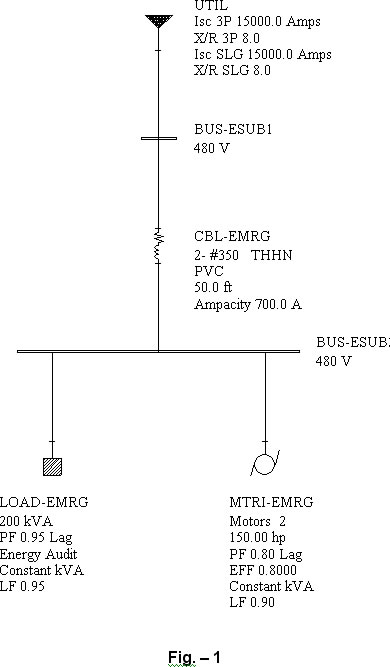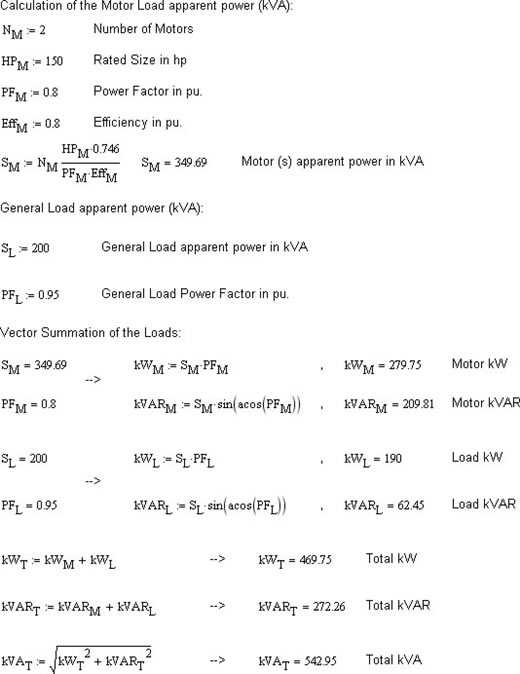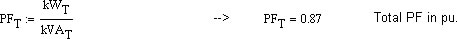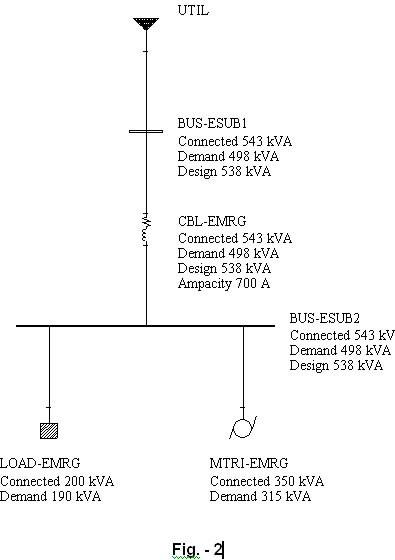Ratings for connected loads are entered as Rated Size of the load in General Load or Induction/Synchronous Motor Subview of the Component Editor (CE).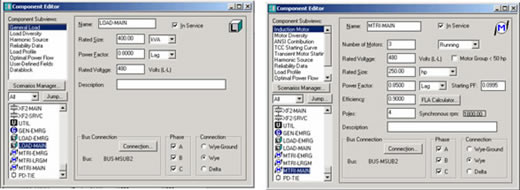If more than one load is connected to a bus then the total connected load will be calculated based on the active and reactive power summation of these loads. The apparent power (kVA) is a vector quantity; therefore vector summation is used for adding the apparent power of the connected loads.

The following example will further emphasize this concept.

Example: Calculate the total Connected Load of Bus-ESUB2 in Fig. 1 then model it in PTW to compare the results.

The solution is presented in the next page and the results match the PTW Demand Load study output displayed in Fig. 2.

Note that you need to connect a source of supply (Swing Bus) to Bus-ESUB1 for getting the Demand Load study results in PTW.